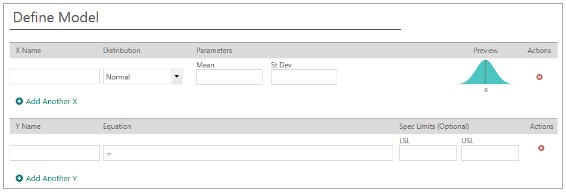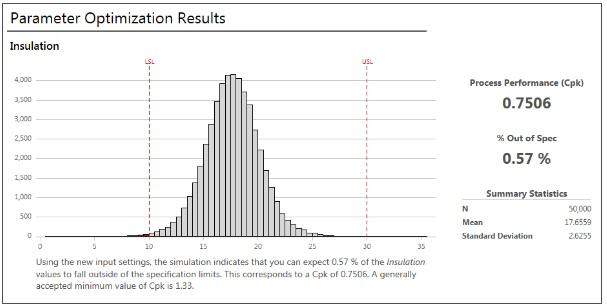# How to perform and apply Monte Carlo simulation?

Monte Carlo simulation is an extension of statistical analysis where simulated data is produced. This method uses repeated sampling techniques to generate simulated data. For instance, a regression model analyzes the effect of independent variables X1 and X2 on dependent variable Y. The regression equation is as follows:

`Y = 0.076 + 0.0054X1 – 0.72X2`

The value of Y can be predicted from the above equation. Moreover, different values of X1 and X2 will give different values of Y. Using this procedure helps generate infinite amount of data. This means variability among different values of X1 and X2 causes variability in its respective values of Y, hence affecting the output. In such cases, Monte Carlo simulation is useful as it simulates the data of X1 and X2. It removes the variability and optimizes the data so that it correctly estimates the value of Y. Therefore, it is broadly a technique to study how a model responds to randomly occurring inputs.

## Process of performing Monte Carlo simulation

There are three main steps in performing Monte Carlo simulation:

1. Perform a regression with ‘N’ inputs (observations of X1 and X2).
2. Run a simulation for each of the ‘N’ inputs. Here simulation refers to the methods to analyze the mean, standard deviation and variance of series X1 and X2 and optimize the same to obtain robust Y.
3. Aggregate and assess the output from the simulations.

## Step by step example of Monte Carlo simulation

In this example first the regression was run. Furthermore, the results from the predicted regression equation were moved to Monto Carlo simulation window (SPPS, Minitab or any other).  This article presents Monte Carlo simulation in Minitab software.

When the Monte Carlo simulation window opens, it presents the below given fields:Image 1: Monte Carlo window in Minitab (Minitab blog website, n.d.)

Here, the model is defined and values of X1, X2 and Y  are expressed as per the estimated regression equation. Write the estimated regression equation. Also, using parameter optimization choose the appropriate lower and upper limit of mean and variation. This will help generate the value of Y as per those conditions (not present in the image below).Image 2: Monte Carlo simulation window defining variable in Minitab software (Minitab blog website, n.d.)

After inserting the values of all variables, click on ‘simulation’. The results will appear in the form of a histogram showing the value of Y with upper limit and lower limit. Here Monte Carlo simulation uses ‘Parameter Optimization’ with minimum mean settings so that number of defects will be minimum. Like in histogram shown in figure below, the value of Y focuses on the mean and carry minimum defects (variability).Figure 3: Parameter optimization results in Minitab (Minitab blog website, n.d.)

## Application of Monte Carlo simulation

Monte Carlo Simulation is useful in probability, mathematical, statistical, physics and financial models. In short, the method is applicable when a variable is expected to be estimated from given random variables based on given equation. It is one of the most popular methods in estimating future stock prices as they follow a random path. Therefore, it can generate thousands and millions of such simulations. Moreover, taking average of these results, one can estimate a reasonable estimation of future stock prices.

## Software supporting Monte Carlo simulation

Software that support Monte Carlo simulation applications are SAS, MATLAB, STATA and SPSS. However, SPSS is less compatible than other software for Monte Carlo simulation. Therefore, other software are more popular for performing the test.

### Indra Giri

Senior Analyst at Project Guru
He completed his Masters in Development Economics from South Asian University, New Delhi. His areas of interest includes various socio development issues like poverty, inequality and unemployment in South Asia. Apart from writing for Project Guru he loves to travel and play football in his spare time.

### Related articles

• How to use an instrumental variable? Instrumental variable is a third variable that estimates causal relationships in the regression analysis when an endogenous variable is present. Instrumental variables are useful when the independent variable in the regression model correlates with the error term in the model.
• How to perform LASSO regression test? In statistics, to increase the prediction accuracy and interpret-ability of the model, LASSO (Least Absolute Shrinkage and Selection Operator) is extremely popular. It is a regression procedure that involves selection and regularisation and was developed in 1989. Lasso regression is an […]
• How to conduct generalized least squares test? In statistics, Generalized Least Squares (GLS) is one of the most popular methods for estimating unknown coefficients of a linear regression model when the independent variable is correlating with the residuals.
• How to apply logistic regression in a case? Machine learning involves solutions to predict scenarios based on past data. Logistic regression offers probability functions based on inputs and their corresponding output.
• How to apply missing data imputation? Missing data is one of the most common problems in almost all statistical analyses. If the data is not available for all the observations of variables in the model, then it is a case of ‘missing data’. Missing data are part of almost all researches. They are also a common problem in most […]
Discussions

1.Debapi Basu
2.Priya